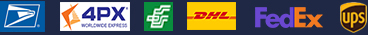•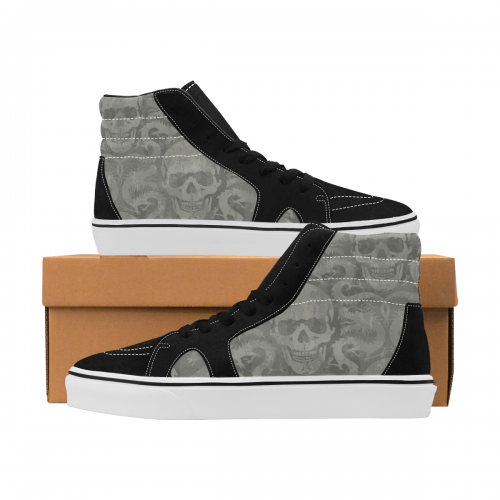•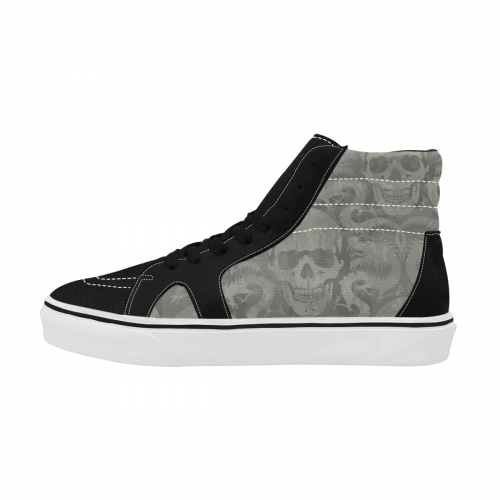•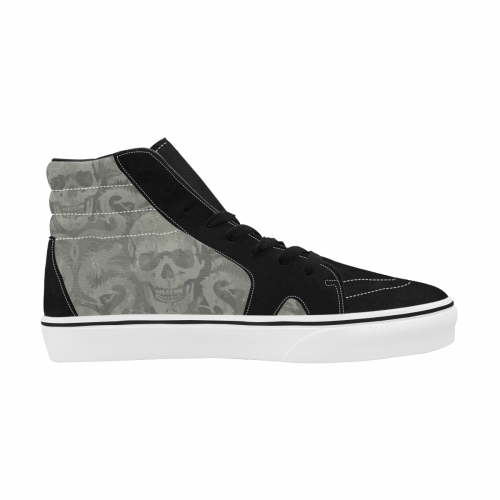•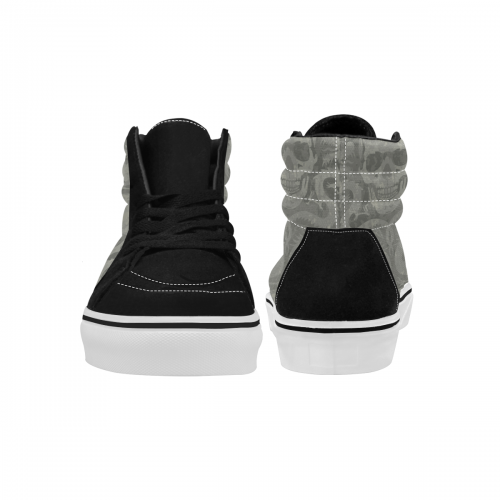### Men's High Top Canvas Shoes (Model E001-1)

\$39.99
Retail Price \$69.99

Partial Shipping Included

•   Black

EUR38 = US6 =UK5 =246mm =9.70inch
EUR39 = US6.5 =UK6 = 253mm = 9.96inch
EUR40 = US7.5 =UK7= 259mm = 10.20inch
EUR41 = US8 =UK7.5 = 266mm =10.47inch
EUR42 = US9 =UK8 =273mm =10.74inch
EUR43 = US9.5 =UK8.5 =279mm = 10.98inch
EUR44 = US10.5 =UK9.5 = 286mm = 11.26inch
EUR45 = US11.5 =UK10.5 = 292mm = 11.50inch

Payment methods we accept: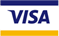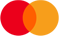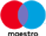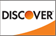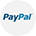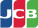We deliver with: